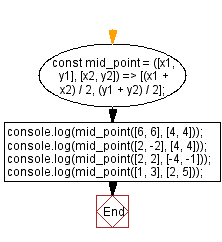# JavaScript - Calculate the midpoint between two points

## JavaScript fundamental (ES6 Syntax): Exercise-267 with Solution

Write a JavaScript program to calculate the midpoint between two pairs of points.

• Destructure the array to get x1, y1, x2 and y2.
• Calculate the midpoint for each dimension by dividing the sum of the two endpoints by 2

Sample Solution:

JavaScript Code:

``````const mid_point = ([x1, y1], [x2, y2]) => [(x1 + x2) / 2, (y1 + y2) / 2];
console.log(mid_point([6, 6], [4, 4]));
console.log(mid_point([2, -2], [4, 4]));
console.log(mid_point([2, 2], [-4, -1]));
console.log(mid_point([1, 3], [2, 5]));
```
```

Sample Output:

```[5,5]
[3,1]
[-1,0.5]
[1.5,4]
```

Flowchart:Live Demo:

See the Pen javascript-fundamental-exercise-267 by w3resource (@w3resource) on CodePen.

Improve this sample solution and post your code through Disqus

What is the difficulty level of this exercise?

Test your Programming skills with w3resource's quiz.

﻿